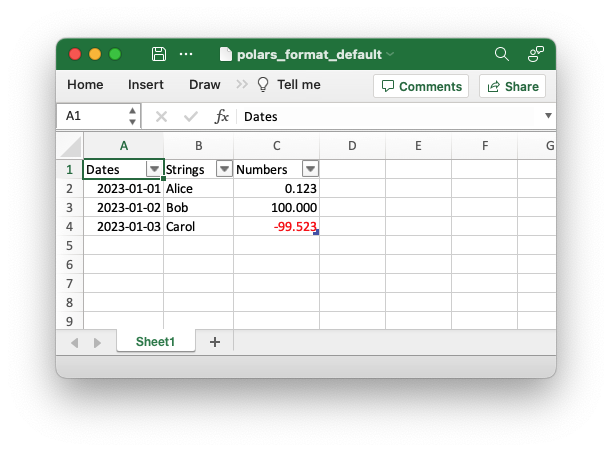# Example: Polars default format example

A simple example of converting a Polars dataframe to an xlsx file with default formatting. See Working with Polars and XlsxWriter for more details.```##############################################################################
#
# A simple example of converting a Polars dataframe to an xlsx file with
# default formatting.
#
# Copyright 2013-2023, John McNamara, jmcnamara@cpan.org
#

from datetime import date
import polars as pl

# Create a Pandas dataframe with some sample data.
df = pl.DataFrame(
{
"Dates": [date(2023, 1, 1), date(2023, 1, 2), date(2023, 1, 3)],
"Strings": ["Alice", "Bob", "Carol"],
"Numbers": [0.12345, 100, -99.523],
}
)

# Write the dataframe to a new Excel file with autofit on.
df.write_excel(workbook="polars_format_default.xlsx", autofit=True)
```